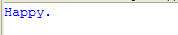Use Scanner to compute an average of the values : Scanner « Development Class « Java

Use Scanner to compute an average of the values

```
/**
*Output:

*/

import java.util.Scanner;

public class MainClass {
public static void main(String args[]) {
Scanner conin = new Scanner(System.in);

int count = 0;
double sum = 0.0;

System.out.println("Enter numbers to average.");

while (conin.hasNext()) {
if (conin.hasNextDouble()) {
sum += conin.nextDouble();
count++;
} else {
String str = conin.next();
if (str.equals("done"))
break;
else {
System.out.println("Data format error.");
return;
}
}
}

System.out.println("Average is " + sum / count);
}
}

```

Related examples in the same category

 1 Read int by using Scanner Class 2 Use Scanner to read user input 3 Count all vowels inputed from keyboard 4 Use Scanner to compute an average of the values in a file 5 Use Scanner to read various types of data from a file 6 Use Scanner to compute an average a list of comma-separated values 7 Demonstrate findInLine() 8 use Scanner to read input that contains several different types of data 9 Letting the user decide when to quit 10 Test the input string in the while condition. 11 This program demonstrates console input. 12 Reading double value from console with Scanner